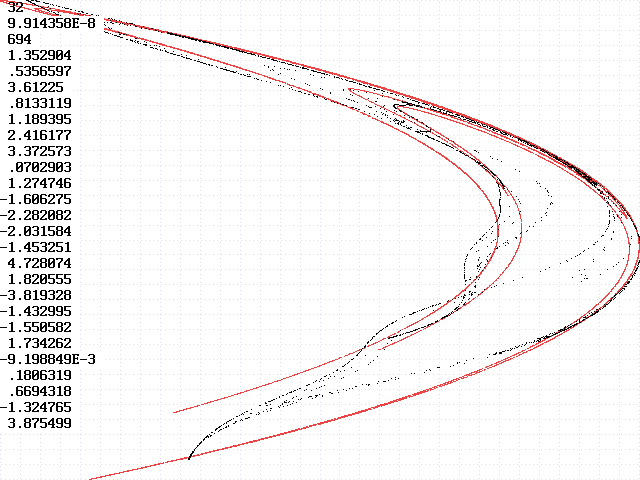# Fitting the Topology of a Strange Attractor

J. C. Sprott
Department of Physics, University of Wisconsin, Madison, WI 53706, USA
February 5, 1998
(Revised April 8, 1999)

Chaotic models designed to fit the short-term dynamics of chaotic data will generally give a poor fit to the long-term dynamics, often not capturing the topology of the attractor even approximately.  The model equations may have unbounded, fixed-point, or periodic solutions even though the fit to the finite chaotic data record is excellent.  This suggests that a better strategy for many purposes might be to optimize the models to fit the shape of the attractor with no consideration of the dynamics that produced it.  To test the feasibility of such a strategy, an artificial neural network was trained to replicate the topology of the Henon attractor.

The attractor was produced by iterating the Henon equations 1000 times with the usual values of a = 1.4 and b = 0.3.  The 2-D xy-space was divided into a 32 x 32 grid of rectangular cells that span the attractor.  The number of data points in each cell was recorded.  A 2-D, single-layer neural network with 6 neurons was then used to produce 1000 points with the same initial conditions, and their distribution among the cells was recorded.  The magnitudes of the differences between the two values were summed over all the cells and used as a measure of the error in the fit.  The neural network parameters were then adjusted to minimize this error using a variant of simulated annealing.  The PowerBASIC source code and compiled version are available.

An example of the screen output from the program is shown below:In red is 32,766 points from the Henon attractor being fit, and the black is 32,766 points from a typical fit to the first 1000 points.  The numbers at the left are the number of grids in each dimension, the size of the region in neural-net connection strengths being searched, the error (maximum of 2000), and the 24 connection strengths.  The fit bears some resemblance to the Henon attractor, but it is clearly not the same and is a bit more complicated.  The solution is trapped in a local minimum in the error, from which there does not appear to be a continuous downhill path to a better solution.  Although the method shows promise, it is apparently quite difficult to replicate the topology of even a simple strange attractor.  The method would almost certainly be even more difficult for a more complicated (higher dimensional) attractor.  Perhaps a better learning algorithm would improve the results.

Back to Sprott's Technical Notes